### Example 3.2 Nonlinear Objective with Constraints Using Repair Mechanism

This example illustrates the use of a repair mechanism to satisfy problem constraints. The problem is to minimize the six-hump camel-back function (Michalewicz 1996, Appendix B):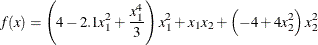whereis within the triangle with vertices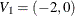,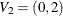, and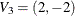. The problem formulation takes advantage of the fact that all feasible solutions can be expressed as a convex combination of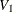,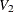, and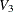in the following form: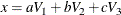In this form,,, andare nonnegative coefficients and satisfy the following linear equality constraint: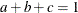Therefore, the solution encoding 'R3' is used with the three elements corresponding to the values of,, and. Note that this strategy can be generalized to any solution domain that can be specified by a convex hull. An additional 'R2' segment is also created to store the correspondingvalue. In the following program, FUNCTION SIXHUMP computes the objective value. Lower and upper bounds of 0 and 1, respectively, are used to ensure that solution elements are nonnegative. Violations of the linear equality constraint are fixed by a simple repair strategy, implemented in FUNCTION SIXHUMP: the sum of the solution elements is computed, and each element is divided by the sum, so that the sum of the new elements is 1. For the special case of all elements equal to 0, equal values are assigned to the solution elements.

proc ga seed = 555;
call SetEncoding('R3R2');
npoints = 3;
array cvxhull[3,2] /nosym ( -2 0
0 2
2 -2 );
/* Objective function */
function sixhump(selected[*],cvxhull[*,*],npoints);
/* Function has global minimum value of -1.0316
* at x = {-0.0898  0.7126} and
*    x = { 0.0898 -0.7126}
*/
array w /nosym;
call dynamic_array(w,npoints);
array x /nosym;
/* make sure that weights add up to 1 */
sum = 0;
do i = 1 to npoints;
sum + w[i];
end;
/* if all weights 0, then reinitialize */
if sum=0 then do;
sum = npoints;
do i = 1 to npoints;
w[i] = 1;
end;
end;
/* re-normalize weights */
do i = 1 to npoints;
w[i] = w[i] / sum;
end;
call WriteMember(selected,1,w);
/* convert weights to x-coordinate form */
x = 0;
x = 0;
do i = 1 to npoints;
x + w[i] * cvxhull[i,1];
x + w[i] * cvxhull[i,2];
end;
/* write out x coordinates to second segment */
call WriteMember(selected,2,x);
/* compute objective value */
r = (4 - 2.1*x**2 + x**4/3)*x**2 + x*x +
(-4 + 4*x**2)*x**2;
return(r);
endsub;
call SetObjFunc('sixhump',0);
array lower /nosym;
array upper /nosym;
call dynamic_array(lower, npoints);
call dynamic_array(upper, npoints);
do i = 1 to npoints;
lower[i] = 0;
upper[i] = 1;
end;
call SetBounds(lower, upper, 1);
array delta /nosym (0.01 0.01 0.01);
call SetMut('delta', 'nchange', 1, 'delta', delta);
call SetMutProb(0.05);
call SetCross('Twopoint', 'alpha', 0.9);
call SetCrossProb(0.8);
call SetSel('tournament', 'size', 2);
call SetElite(3);
call Initialize('DEFAULT', 200);
call ContinueFor(200);
run;


Note that this problem uses the standard genetic operators and default initialization, even though they generate solutions that violate the constraints. This is possible because all solutions are passed into the user objective function for evaluation, where they are repaired to fit the constraints. The output is shown in Output 3.2.1.

Output 3.2.1: Nonlinear Objective with Constraints Using Repair Mechanism

PROC GA Optimum Values

Objective
-1.031617314

Solution
Segment Element Value
1 1 0.2439660392
1 2 0.5561415112
1 3 0.1998924497
2 1 -0.088147179
2 2 0.712498123

This objective function has a global minimum at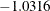, at two different points: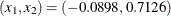and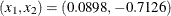. The genetic algorithm can converge to either of these minima, depending on the random number seed set by the SEED= option.